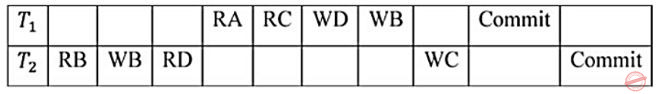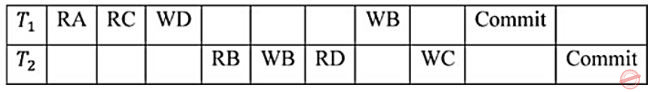October 9, 2023
October 9, 2023
October 9, 2023
###### Logical-Reasoning
October 9, 2023
 Question 10

Consider a schedule of transactions T1 and T2:Here, RX stands for “Read(X)” and WX stands for “Write(X)”. Which one of the following schedules is conflict equivalent to the above schedule?

 ABCDQuestion 10 Explanation:
• Two schedules are said to be conflict equivalent, if conflict operations in both the schedules are executed in the same order.
• First, let’s list the conflict operations of each of the schedule given in the options and compare with the conflict operations of schedule which is given in the question.
Given schedule:Conflict operations:
R2(B) → W1(B)
W2(B) → W1(B)
R1(C) → W2(C)
R2(D) → W1(D)

Option(1):Conflict operations:
R1(C) → W2(C)
W1(D) → R2(D)
W1(B) → R2(B)
W1(B) → W2(B)

Option(2):Conflict operations:
R2(B) → W1(B)
W2(B) → W1(B)
R2(D) → W1(D)
R1(C) → W2(C)

Option(3):Conflict operations:
R2(B) → W1(B)
W2(B) → W1(B)
R2(D) → W1(D)
W2(C) → R1(C)

Option(4):Conflict operations:
R1(C) → W2(C)
W1(D) → R2(D)
R2(B) → W1(B)
W2(B) → W1(B)

The conflict operations in the option (2) and given schedule are appearing in the same sequence order, so option (2) is the answer.

Question 10 Explanation:
• Two schedules are said to be conflict equivalent, if conflict operations in both the schedules are executed in the same order.
• First, let’s list the conflict operations of each of the schedule given in the options and compare with the conflict operations of schedule which is given in the question.
Given schedule:Conflict operations:
R2(B) → W1(B)
W2(B) → W1(B)
R1(C) → W2(C)
R2(D) → W1(D)

Option(1):Conflict operations:
R1(C) → W2(C)
W1(D) → R2(D)
W1(B) → R2(B)
W1(B) → W2(B)

Option(2):Conflict operations:
R2(B) → W1(B)
W2(B) → W1(B)
R2(D) → W1(D)
R1(C) → W2(C)

Option(3):Conflict operations:
R2(B) → W1(B)
W2(B) → W1(B)
R2(D) → W1(D)
W2(C) → R1(C)

Option(4):Conflict operations:
R1(C) → W2(C)
W1(D) → R2(D)
R2(B) → W1(B)
W2(B) → W1(B)

The conflict operations in the option (2) and given schedule are appearing in the same sequence order, so option (2) is the answer.